Forum Soutien Scolaire - Physique Chimie- Filtre optionnel -
Recherche d'un mot :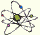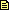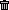20856 -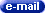de rajesh , 15 ans (india) - 2021-05-27 Physique Chimie : "I need help with homework"

is someone smart enough to solve this? i asked my brother ha cant help me...

An athlete throws the hammer at an angle of about 39.95 ° reaching the
distance of 86.74 m. The tool consists of a sphere weighing 7.26 kg and radius
0.06 cm and a chain and a handle for a total length of 1.195 m. The height from
from which the tool started was about 1.7 meters. The total turning radius is 1.95 m.
1. Calculate how fast the hammer was thrown
2. Calculate the angular speed of rotation of the athlete at the moment of the throw.
3. Calculate the centripetal force exerted by the athlete immediately before the release (assuming
a uniform circular motion and neglecting the force of gravity)
4. Write the trajectory equation and draw its graph. Calculate the coordinates of the
point of greatest height.
5. Assuming that once launched, the hammer rotates on itself at the angular speed of
0.2 rounds per second calculate the kinetic energy and potential energy at the point of maximum altitude.
Note: the moment of inertia of the hammer is 0.2 kg * m2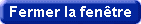Forum Soutien Scolaire - Physique Chimie - (c) Etudiants du Monde / Students of the World
pour toute remarque / question, merci de contacter le webmaster :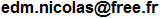www.StudentsOfTheWorld.info